# Arc tangent function

This article is about a particular function from a subset of the real numbers to the real numbers. Information about the function, including its domain, range, and key data relating to graphing, differentiation, and integration, is presented in the article.
View a complete list of particular functions on this wiki
For functions involving angles (trigonometric functions, inverse trigonometric functions, etc.) we follow the convention that all angles are measured in radians. Thus, for instance, the angle of$90\,^\circ$ is measured as$\pi/2$.

## Definition

The arc tangent function, denoted$\arctan$ or$\tan^{-1}$, is a function defined as follows: for$x \in \R$,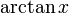$\arctan x$ is the unique number$y$ in the open interval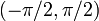$(-\pi/2,\pi/2)$ such that$\tan y = x$.

Equivalently, the arc tangent function is the inverse function to the restriction of the tangent function to the interval$(-\pi/2,\pi/2)$.

## Key data

Item Value
default domain all real numbers, i.e., all of$\R$
range the open interval$(-\pi/2,\pi/2)$, i.e., the set$\{y \mid -\pi/2 < y< \pi/2 \}$
no absolute minimum or absolute maximum, because the extremes are attained asymptotically at infinity.
local minimum values and points of attainment no local minimum values
local maximum values and points of attainment no local maximum values
points of inflection (both coordinates)$(0,0)$ (the origin) only
horizontal asymptotes The line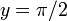$y = \pi/2$ as$x \to \infty$
The line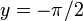$y = -\pi/2$ as$x \to -\infty$
derivative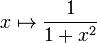$x \mapsto \frac{1}{1 + x^2}$ (see #First derivative)
second derivative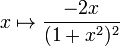$x \mapsto \frac{-2x}{(1 + x^2)^2}$ (see #Second derivative)
higher derivatives The$n^{th}$ derivative is a rational function of$x$ where the denominator degree is$n + 1$ more than the numerator degree.
antiderivative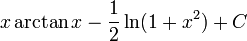$x \arctan x - \frac{1}{2}\ln(1 + x^2) + C$. We use integration by parts (see #Integration).
higher antiderivatives The function can be antidifferentiated any number of times in terms of elementary functions.

## Graph

Here is a graph of$\arctan$ from a zoomed out position, where the horizontal asymptotes are clear.

Here is a more close-up version, with the tangent line through the origin (the line$y = x$) drawn, indicating that the origin is a point of inflection for the graph:

## Differentiation

### First derivative

WHAT WE USE: inverse function theorem and tangent function#First derivative, which in turn relies on sine function#First derivative, cosine function#First derivative, and quotient rule for differentiation

We use the inverse function theorem, and the fact that the derivative of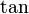$\tan$ is$\sec^2$.

By the inverse function theorem, we have: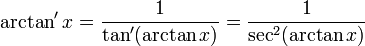$\arctan' x = \frac{1}{\tan'(\arctan x)} = \frac{1}{\sec^2(\arctan x)}$

If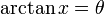$\arctan x = \theta$, then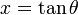$x = \tan \theta$ and we get:$\! \sec^2\theta = 1 + \tan^2\theta = 1 + x^2$

Plugging this into the above, we get:$\arctan' x = \frac{1}{1 + x^2}$

### Second derivative

WHAT WE USE: chain rule for differentiation and differentiation rule for power functions

The second derivative is given as:$\arctan'' x = \frac{d}{dx} \arctan'x = \frac{d}{dx}\left(\frac{1}{1 + x^2}\right) = \frac{d}{dx}[(1 + x^2)^{-1}] = (2x)(-1)(1 + x^2)^{-2} = \frac{-2x}{(1 + x^2)^2}$

### Higher derivatives

For higher derivatives, we use the quotient rule for differentiation, combined with the chain rule for differentiation to deal with powers of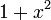$1 + x^2$.

## Integration

### First antiderivative

We can integrate this using the inverse function integration method, and obtain: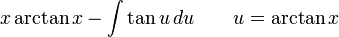$x \arctan x - \int \tan u \, du \qquad u = \arctan x$

This becomes:$x \arctan x + \ln|\cos u| + C$

We have$\ln|\cos u| = (1/2)\ln(\cos^2 u) = -1/2\ln(\sec^2 u) = (-1/2)\ln(1 + x^2)$, and we get:$x \arctan x - \frac{1}{2}\ln(1 + x^2) + C$

More explicitly, we can do the integration using integration by parts taking$\arctan x$ as the part to differentiate and$1$ as the part to integrate: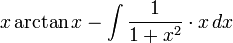$x \arctan x - \int \frac{1}{1 + x^2} \cdot x \, dx$

For the second integration, we integrate using the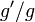$g'/g$ formulation to get$(1/2)\ln(1 + x^2)$.

### Higher antiderivatives

The function can be antidifferentiated any number of times using integration by parts. The reason for this is that the derivative of the function is a rational function, and rational functions can be repeatedly integrated within elementarily expressible functions.

All the antiderivatives can be expressed in the form: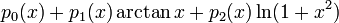$p_0(x) + p_1(x)\arctan x + p_2(x)\ln(1 + x^2)$

where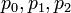$p_0,p_1,p_2$ are polynomial. Note that$p_0$ is ambiguous up to addition of polynomials of degree$n - 1$ if we are integrating$n$ times.

### Higher antiderivatives

The function can be antidifferentiated any number of times using integration by parts.

## Power series and Taylor series

### Computation of power series

The power series for the function about 0 can be obtained as follows.

We know that for the function$1/(1 + x^2)$, we have the power series: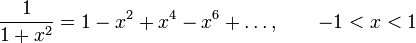$\frac{1}{1 + x^2} = 1 - x^2 + x^4 - x^6 + \dots, \qquad -1 < x < 1$

Integrating with a definite integral, we get:$\! \int_0^x \frac{1}{1 + t^2} \, dt = x - \frac{x^3}{3} + \frac{x^5}{5} - \frac{x^7}{7} + \dots, -1 < x < 1$

The left side is$[\arctan t]_0^x = \arctan x - \arctan 0 = \arctan x$, so we get: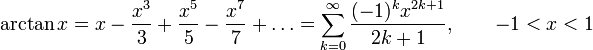$\arctan x = x - \frac{x^3}{3} + \frac{x^5}{5} - \frac{x^7}{7} + \dots = \sum_{k=0}^\infty \frac{(-1)^kx^{2k+1}}{2k + 1}, \qquad -1 < x < 1$

By the alternating series theorem, we note that the power series on the right converges for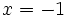$x = -1$ and for$x = 1$, so by Abel's theorem, we conclude that it converges to$\arctan$ of the respective inputs. We thus get:$\arctan x = x - \frac{x^3}{3} + \frac{x^5}{5} - \frac{x^7}{7} + \dots = \sum_{k=0}^\infty \frac{(-1)^kx^{2k+1}}{2k + 1}, \qquad -1 \le x \le 1$